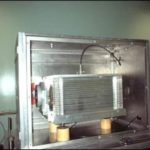# Air wall ionization chambersIn the field of low and medium energy X-ray dosimetry, the air chambers correspond to the primary references for the dosimetry quantity of interest (kerma in air, dose in air or water).

Their principle of operation is relatively simple: the electrical signal delivered by this type of detector depends directly on the energy deposited in a known volume of air, which allows to go back to the reference kerma.

Each chamber must be adapted to the energy range to be measured. Thus, each X-ray generator in the laboratory is equipped with its own reference air chamber:

 Chamber reference CE98 MD03 WK07 WK06 Facility Mammograph Radiodiagnosis Phase X Gulmay 160 kV Seifert 320 kV High voltage range (kV) 20 – 49 40 – 150 10 – 100 20 – 320 Electric field strength (V/cm) 500 345 254 278 Opening diameter (cm) 0.505 0.997 0.5009 1.0074 Measuring volume (cm³) 0.3391 3.781 0.4028 4.7827 Collector electrode width (cm) 1.693 4.844 2.0445 6.0004 Distance between electrodes (cm) 5.0 14.5 11.8 18.0 Air thickness for electronic balance (cm) 6.05 20.4 7.2 31.8

### Principle of reference kerma measurement and calibration of an ionization chamber

The kerma in air can be calculated from the measurement of the charge Q created in an air chamber during the interaction of the photons with the air of the detection volume V. The general expression of kerma is given:

$$K_{air} = \displaystyle{\frac{Q}{\rho\cdot V}\cdot\frac{W_{air}}{e}\cdot\frac{1}{(1-g_{air})}\cdot\prod ki}$$

where

Q is the measured charge in coulomb (C)
V is the measurement volume (m3)
ρ is the atomic mass of air (kg.m-3)
gair [ICR80] is a fraction of the initial kinetic energy of electrons produced by the interaction of photons in matter that is not absorbed in the air and converted into photons (Bremsstrahlung radiation)
Πki is the product of the correction factors to be applied.

The method of calibrating a cavity ionization chamber is to first measure the kerma in air of a reference beam using an air wall chamber and then place the chamber to be calibrated under the same irradiation conditions (distance, photon fields, flow rate, beam quality). The charge Qclient generated in the chamber volume by the incident X-ray beam is then measured using an electrometer.

The calibration coefficient Nk of the chamber can then be written:

$$N_k = \displaystyle{\frac{K_{air}}{Q_{client}}}$$

Material calibrated using these references:

→ Active measurement systems: cavity ionization chambers, semiconductor detectors (operational dosimeters)

→ Passive measurement systems: TLD dosimeters, OSL dosimeters, radio films

# Our job: metrology

## dosimetry

Implemented methods for the establishment of national references must be adapted to the radiation type and its intensity. They are based on measurement technics such as calorimetry, ionometry and chemical dosimetry.